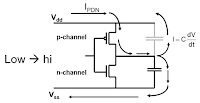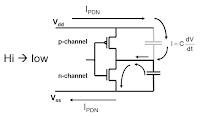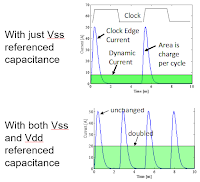## You need to test, we're here to help.

You need to test, we're here to help.

## 24 January 2018

### Making On-Die Power-Rail Measurements (Part II)Figure 1: When switching from low to high, PDN noise flows from the Vdd rail through to the Vss rail
Now that we're ready to begin some on-die power-rail measurements, it's a good idea to step back for a moment and anticipate what these measurements should show us. What actually happens on the die when CMOS gates are switching on and off? What should we expect to see on the on-die power rails? And what happens at the clock edges?

When the P-channel device (or any gate on the die, for that matter) switches from logic low to logic high, noise from the power-distribution network (PDN) on the die will flow from the Vdd/Vcc rail all the way through to the Vss rail (Figure 1). This is because the capacitance of the output line is referenced to the capacitances of both rails when the device switches from low to high.Figure 2: When switching from high to low, PDN noise still flows through the impedance of the PDN
What happens when the device switches from high to low (Figure 2)? It's logical to think that it would simply discharge the capacitance across the N-channel gate and that current flow would be local to that loop. But on the die (and sometimes also on the board), that output capacitance is also referenced to Vdd/Vcc through the P-channel capacitance. Thus, when the output line switches from logic high to logic low, yes, we discharge the current in that capacitor across the N-channel gate. But we also have a voltage drop through the P-channel capacitor, and the dV/dT means that current is flowing there, too.Figure 3: Expect to see asymmetry in the current draw as clock edges rise and fall.
So even when we go from logic high to logic low, we will still have PDN current flowing through the PDN's impedance. That, in turn, means that we will see current flowing on both edges of the clock.

In looking at both edges of the clock, we expect to find that when the clock turns on, all the gates are typically edge-switched. Generally speaking, depending on the logic design, when the clock edge rises, the gates are switched, and when the clock edge falls, the gates are latched. In latching, there should be less current draw than in switching. So even though we get PDN current flowing between Vdd/Vcc and Vss on both clock edges, there should be an asymmetry in that current flow (Figure 3).

That sums up our expectations of what we should see on the on-die power rails. Next time, we'll look at the actual measurement results.

Previous posts in this series:

Understand RF Pickup When Measuring Power Rails
How 10X Attenuating Probes Kill Signal-To-Noise Ratio
Bandwidth vs. Current Load in Power-Rail Measurements
Power-Rail Noise: Small Signal, Big DC Offset
Setting the Stage for On-Die Power-Rail Measurements
Measuring Shared On-Die Power Rails
Making On-Die Power-Rail Measurements (Part I)